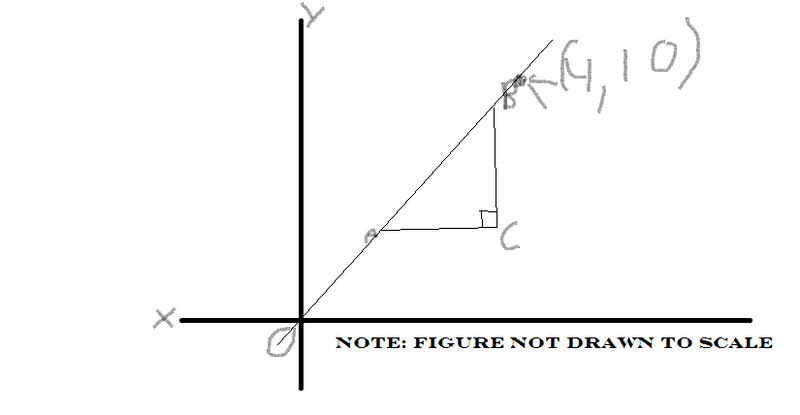# Practicing for SAT Math and had my first four part miserable failure sequence

For these four practice problems, I had little idea what the hell to do (plugging in seemed worthless), could anyone help? Sorry if this isn't what the board is suppose to be used for. Answers in spoiler tags.

If k and h are constants and x2+kx+7 is equivalent to (x+1)(x+h), what is the value of k?
8
-----------------------------------------------------------------In the figure above, if the legs of the triangle ABC are parallel to the axes, which of the following could be the lengths of the sides of triangle ABC?

2,5,sqrt 29
2,5,7
3,3,3 sqrt2
3,4,5
4,5, sqrt41

2,5 sqrt 29
-------------------------------------------------------

Let the function f be defines as f(x)=2x-1. If 1/2f(sqrt t)=4, what is the value of t?

3/sqrt 2
7/2
9/2
49/4
81/4

81/4
----------------------------------------------------------

If k is a positive integer, which of the following must represent an even integer twice the value of the odd integer?

2k
2k+3
2k+4
4k+1
4k+2

4k+2

It would be a great help if anyone could even point me into the right direction on these

gb7nash
Homework Helper
1) Multiply out (x+1)(x+h) by FOILing and equate it to x2+kx+7. What's the first conclusion you must make? What's the second conclusion?

2) The most important thing here is the slope of that line. Take into account that the slope of the line is rise/run. What's a possibity for the value of rise? run? (looking at the answers) Once you have those two values, how do you find the hypotenuse?

3) Judging from the answer, it looks like you mean (1/2)f(sqrt t)= 4, not 1/[2f(sqrt t)]= 4. The use of parentheses here is very important. If f(x) = 2x - 1, what's f(sqrt(t))? Once you have this, plug it into (1/2)f(sqrt t)= 4 and solve for t.

4) Something is missing from this problem, but I think what you meant to say is define an odd integer (call it j) as j = 2k+1, where k is a positive integer. If this is the case, how do we represent twice the odd integer?

If k and h are constants and x2+kx+7 is equivalent to (x+1)(x+h), what is the value of k?
Multiply out (x+1)(x+h) and the answer is obvious.

In the figure above, if the legs of the triangle ABC are parallel to the axes, which of the following could be the lengths of the sides of triangle ABC?
There are two conditions here. First, the ratio of the two legs has to equal 0.4, because the line goes through the origin and (4, 10). The second condition is the Pythagorean theorem.
-------------------------------------------------------

Let the function f be defines as f(x)=2x-1. If 1/2f(sqrt t)=4, what is the value of t?
This one CAN be solved just by plugging in: plug the definition of f into the equation, then solve for t.

If k is a positive integer, which of the following must represent an even integer twice the value of the odd integer?
For any integer k, 2k is even, and 2k+1 is odd. To be twice the value of an odd integer, just double that.

Multiply out (x+1)(x+h) and the answer is obvious.

There are two conditions here. First, the ratio of the two legs has to equal 0.4, because the line goes through the origin and (4, 10). The second condition is the Pythagorean theorem.
-------------------------------------------------------

This one CAN be solved just by plugging in: plug the definition of f into the equation, then solve for t.

For any integer k, 2k is even, and 2k+1 is odd. To be twice the value of an odd integer, just double that.

2. Got it, 2,5 sqrt 29 is the only one that fits the 4:10 ratio

3. Didn't realize it was that obvious

4. Okay the makes sense.

Still no idea what to do on the first one

Still no idea what to do on the first one
(x+1)(x+h)
x(x+h) + 1(x+h)
x^2 + xh + x + h
x^2 + x(h+1) + h

Does that help?

I still cannot figure it out.

I still cannot figure it out.
Sure you can. Compare these two expressions:

x2 + (h+1)x + h

x2 + kx + 7

What values of h and k make them the same?

I don't see why there is a limit to what h and k can equal.

I don't see why there is a limit to what h and k can equal.
You may be overthinking this a little. Just LOOK at the expressions

x2 + (h+1)x + h

x2 + kx + 7

Don't they look similar to you? What would it take to make them look identical? (And you know the answer, too -- try plugging that in and see what happens.)

okay I get it now

Char. Limit
Gold Member
It might help if you know that for two polynomials (of equal degree), for the two polynomials to be equal for every x, each of the coefficients must be equal for every x. Your two polynomials are x2+kx+7 and x2+(h+1)x+h. Setting these two equal, with x2+kx+7=x2+(h+1)x+h, we can equate the coefficents of every power of x:

1=1 (the two coefficients of x2)
k=h+1 (the two coefficients of x)
7=h (the two coefficients of the constant term x0)

From there it's easy to conclude that k=8.

It might help if you know that for two polynomials (of equal degree), for the two polynomials to be equal for every x, each of the coefficients must be equal for every x. Your two polynomials are x2+kx+7 and x2+(h+1)x+h. Setting these two equal, with x2+kx+7=x2+(h+1)x+h, we can equate the coefficents of every power of x:

1=1 (the two coefficients of x2)
k=h+1 (the two coefficients of x)
7=h (the two coefficients of the constant term x0)

From there it's easy to conclude that k=8.

O_O This is great. Thank you.